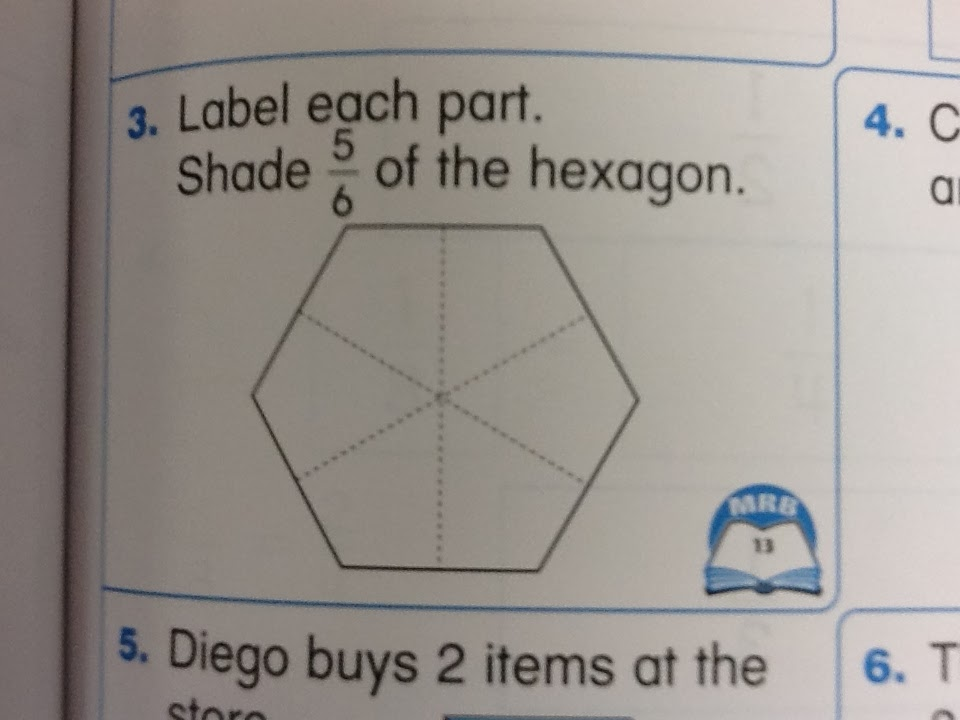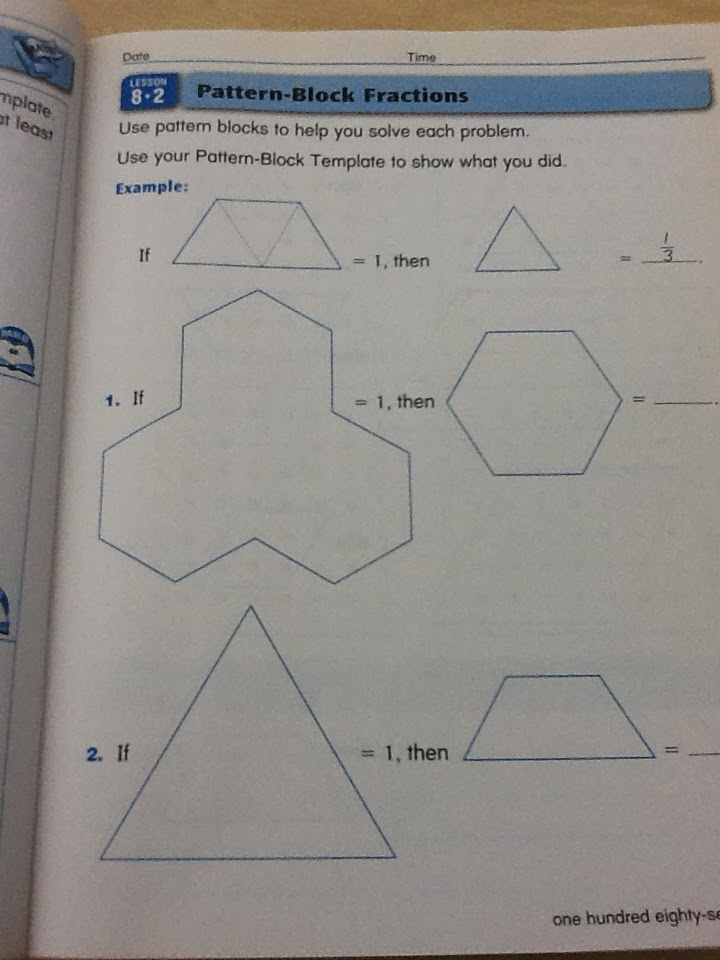Wednesday, January 29, 2014

Lots of questions

I've been carrying around a copy of the Common Core math standards for several years now.  It's all annotated and dog-eared from use doing summer curriculum revisions, and from all the times I've been in classrooms, seen something taught (or taught something myself), and asked: was that really necessary?  I thought I knew them well, but you learn something new every day.
Monday we had a math specialists meeting and one of our tasks was to check and revise our grades 1-5 end- of- year tests.  While revising and aligning the grade 2 assessment I came across this:

• CCSS.Math.Content.2.G.A.3 Partition circles and rectangles into two, three, or four equal shares, describe the shares using the words halves, thirds, half of, a third of, etc., and describe the whole as two halves, three thirds, four fourths. Recognize that equal shares of identical wholes need not have the same shape.

I did a double-take, and checked the rest of the grade 2 standards.  This was the only mention of anything related to fractions, it was appearing in the geometry domain, and there was no formal symbolism.  No numbers, no numerator and denominator, no fraction bar, only descriptive language.  Words.
I tracked back to grade 1 and found this, again in the geometry domain.

• CCSS.Math.Content.1.G.A.3 Partition circles and rectangles into two and four equal shares, describe the shares using the words halvesfourths, and quarters, and use the phrases half of,fourth of, and quarter of. Describe the whole as two of, or four of the shares. Understand for these examples that decomposing into more equal shares creates smaller shares.
Again, describe using words.  Then I looked at grade 3 and found this:

• CCSS.Math.Content.3.NF.A.1 Understand a fraction 1/b as the quantity formed by 1 part when a whole is partitioned into b equal parts; understand a fraction a/b as the quantity formed by a parts of size 1/b.
• CCSS.Math.Content.3.NF.A.2 Understand a fraction as a number on the number line; represent fractions on a number line diagram.
• CCSS.Math.Content.3.NF.A.2a Represent a fraction 1/b on a number line diagram by defining the interval from 0 to 1 as the whole and partitioning it into b equal parts. Recognize that each part has size 1/b and that the endpoint of the part based at 0 locates the number 1/b on the number line.
• CCSS.Math.Content.3.NF.A.2b Represent a fraction a/b on a number line diagram by marking off a lengths 1/b from 0. Recognize that the resulting interval has size a/b and that its endpoint locates the number a/b on the number line.
• CCSS.Math.Content.3.NF.A.3 Explain equivalence of fractions in special cases, and compare fractions by reasoning about their size.
• CCSS.Math.Content.3.NF.A.3a Understand two fractions as equivalent (equal) if they are the same size, or the same point on a number line.
• CCSS.Math.Content.3.NF.A.3b Recognize and generate simple equivalent fractions, e.g., 1/2 = 2/4, 4/6 = 2/3. Explain why the fractions are equivalent, e.g., by using a visual fraction model.
• CCSS.Math.Content.3.NF.A.3c Express whole numbers as fractions, and recognize fractions that are equivalent to whole numbers. Examples: Express 3 in the form 3 = 3/1; recognize that 6/1 = 6; locate 4/4 and 1 at the same point of a number line diagram.
• CCSS.Math.Content.3.NF.A.3d Compare two fractions with the same numerator or the same denominator by reasoning about their size. Recognize that comparisons are valid only when the two fractions refer to the same whole. Record the results of comparisons with the symbols >, =, or <, and justify the conclusions, e.g., by using a visual fraction model.
1 Grade 3 expectations in this domain are limited to fractions with denominators 2, 3, 4, 6, 8.

So in third grade fractions has its own domain, with 7 separate standards, that treat fractions in a very formal way.

So this has got me wondering:

When we teach "fraction readiness" to first and second graders, should we stay away from formal symbolism altogether?  Was that the intention of the common core writers?  Would focusing exclusively on the words improve a child's understanding of fractions, and how might this be related to what Dan is talking about here ? (read the quotes he pulled out from Pimm's book)

Are exercises like this:From our grade 1 math journal

and this:Grade 2
and this:More from grade 2

And even if children are able to complete these pages (and others like them) correctly, does that indicate real understanding, or is it an example of Gardner's "correct answer compromise"
And for very practical purposes: What has to happen in grade 3 to help children make what seems to be an enormous leap towards a very formal understanding of fractions?
Lots of questions.  Any thoughts?